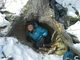Scroll

# Question

If abc/def = a/d * b/e * c/f, then why doesn't (a * b)/c = (a/c)(b/c)? The quiz solution says (a * b)/c = (a/c)b...but shouldn't b have a denominator of c?

Dividing by a value is the same as multiplying by its reciprocal. So dividing by 2 is the same as multiplying by (1/2), or for this problem, dividing by c is the same as multiplying by (1/c). One way you could think about the b/c term is to split it up with parentheses into a set of multiplications:

b/c = (a) * (b) * (1/c)

Since you can re-order multiplications, all these are equivalent statements:

(a)(b)(1/c) = (a)(1/c)(b) = (a/c) * (b)

If you want, we can try plugging in some numbers to make sure that (a * b)/c = (a/c) * b

For example, let's try a = 3, b = 4, and c = 6

(a * b)/c = (3 * 4)/6 = 2

(a/c) * b = (3/6) * 4 = 2

2 = 2

However, for ab/c=(a/c)(b/c), we can't separate out both (a/c) and (b/c). Let's try it with the same numbers above:

a = 3, b = 4, and c = 6

(a * b)/c = (3 * 4)/6 = 2

(a/c)(b/c) = (3/6)(4/6) = (1/2) * (2/3) = 1/3

2 does not equal 1/3

When you simplify fractions, there are a few rules you have to stick to regarding numerators and denominators, the following video lessons may be of help:

operations with fractions

fraction properties

http://gre.magoosh.com/questions/2619

•